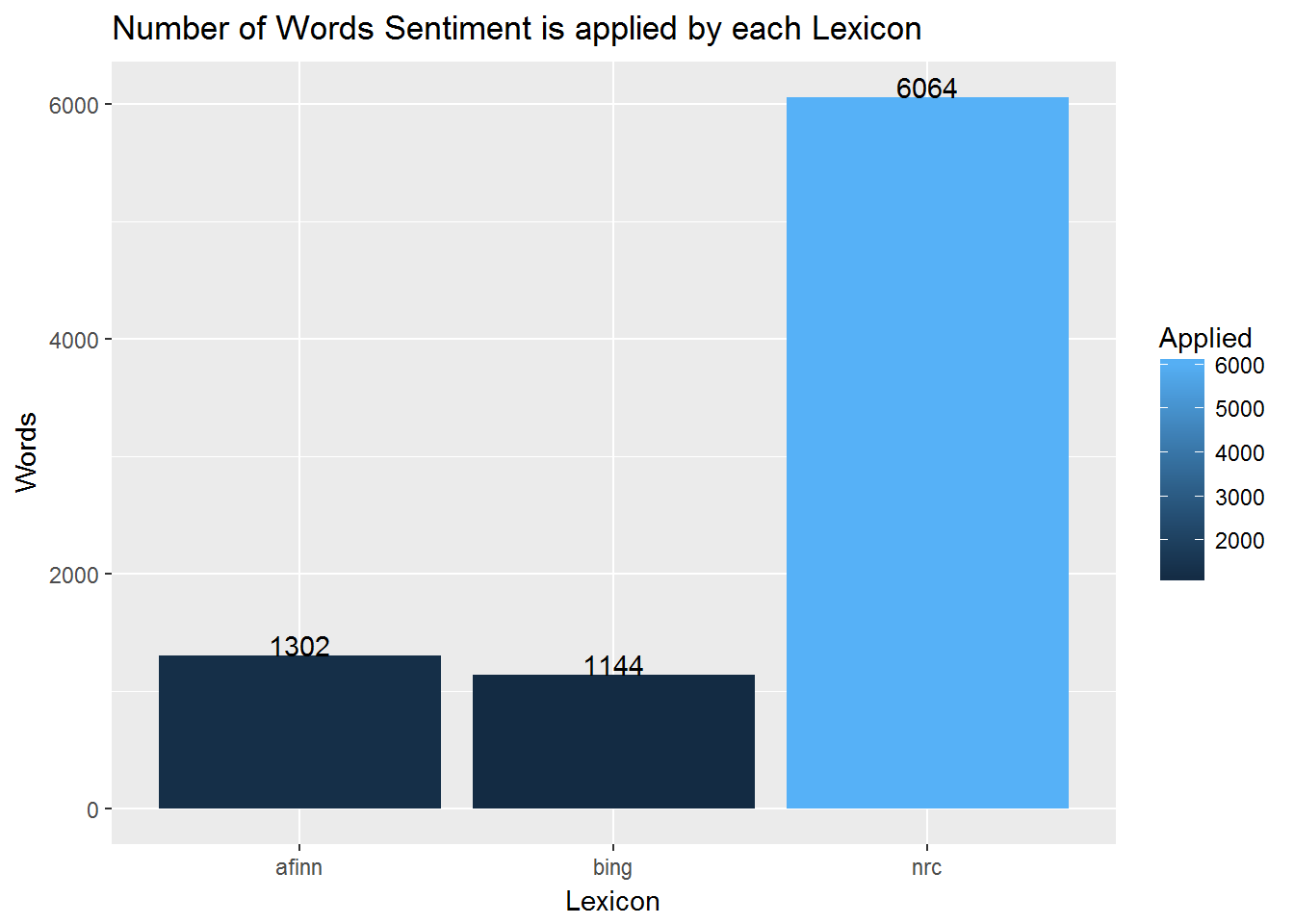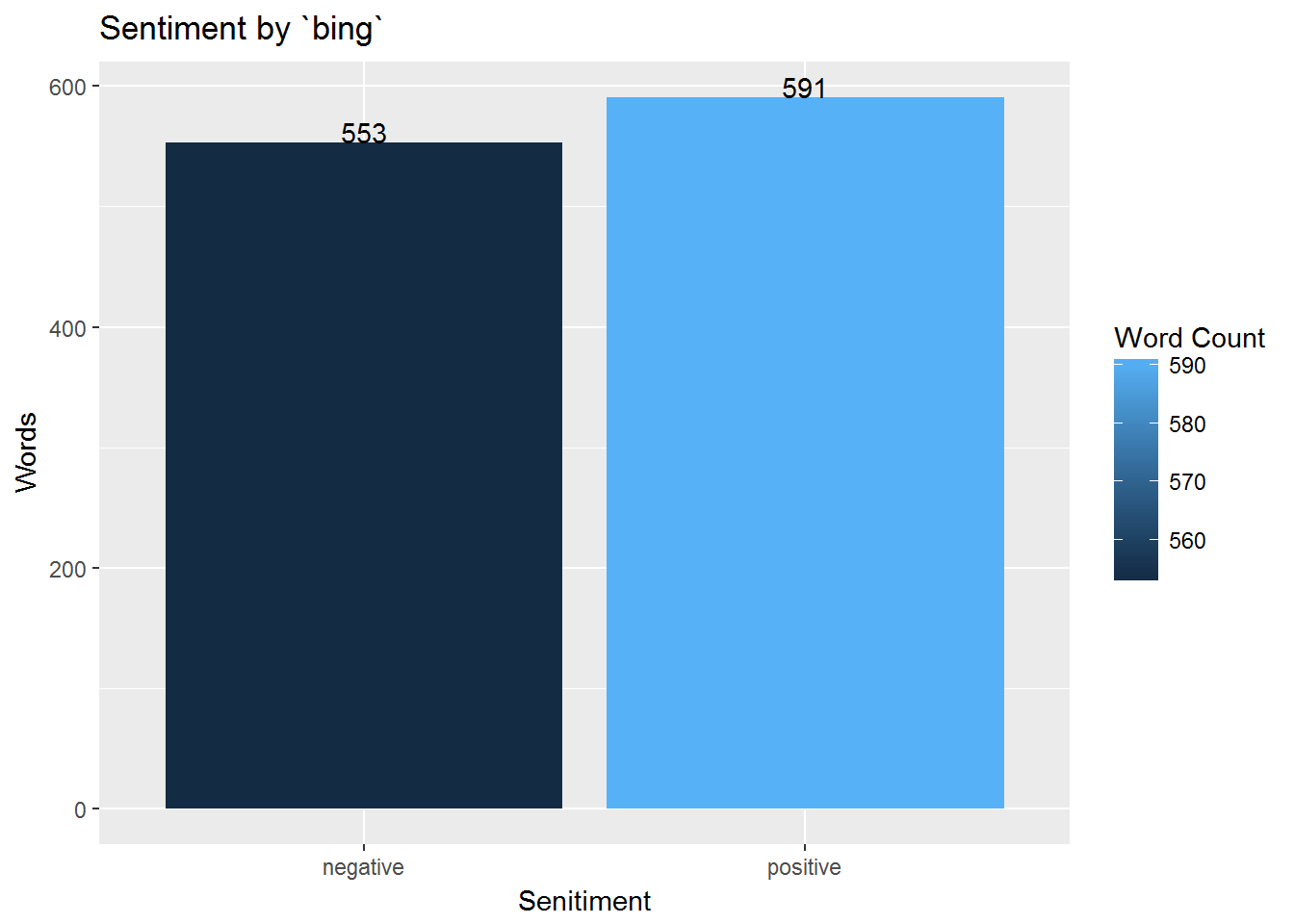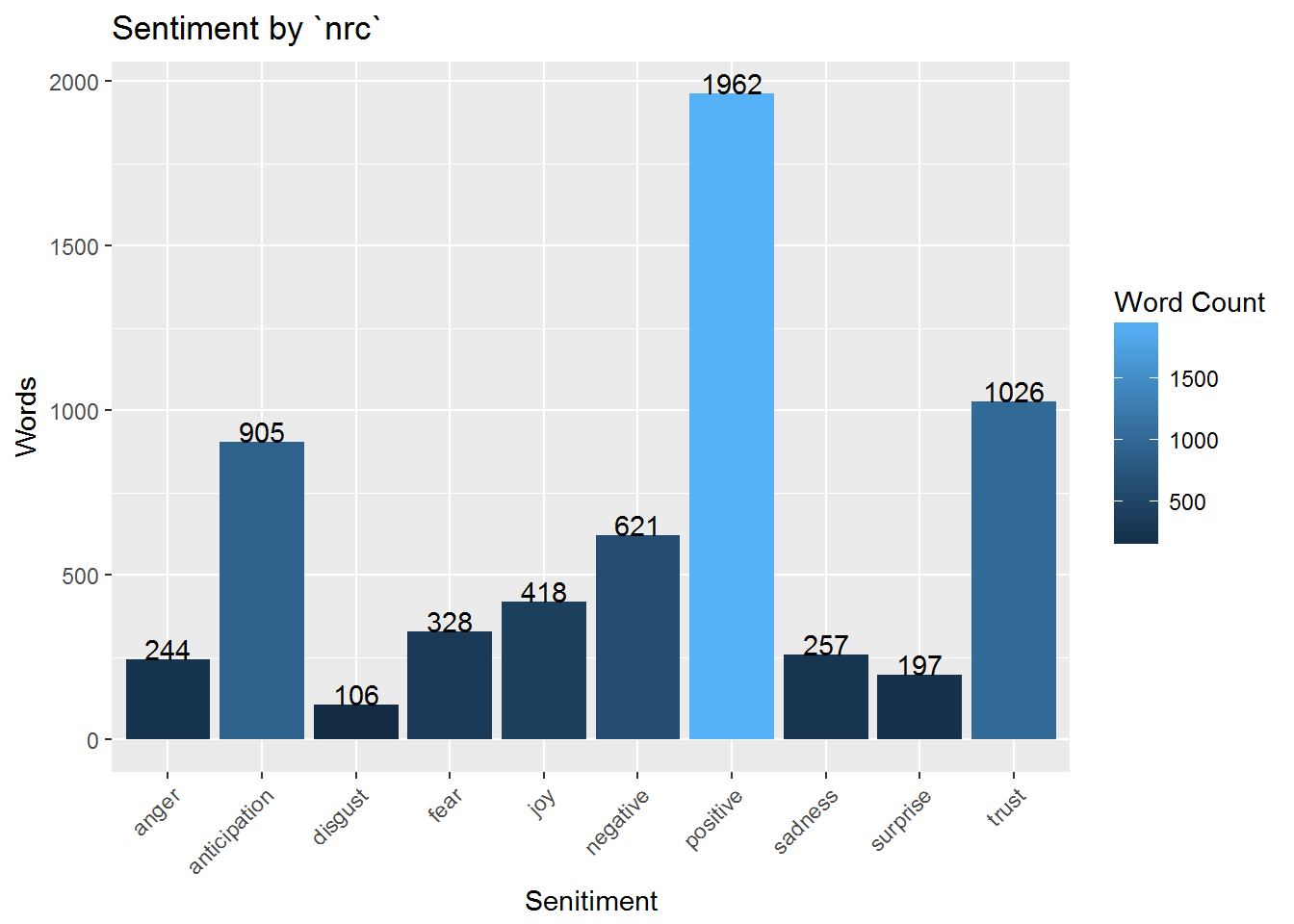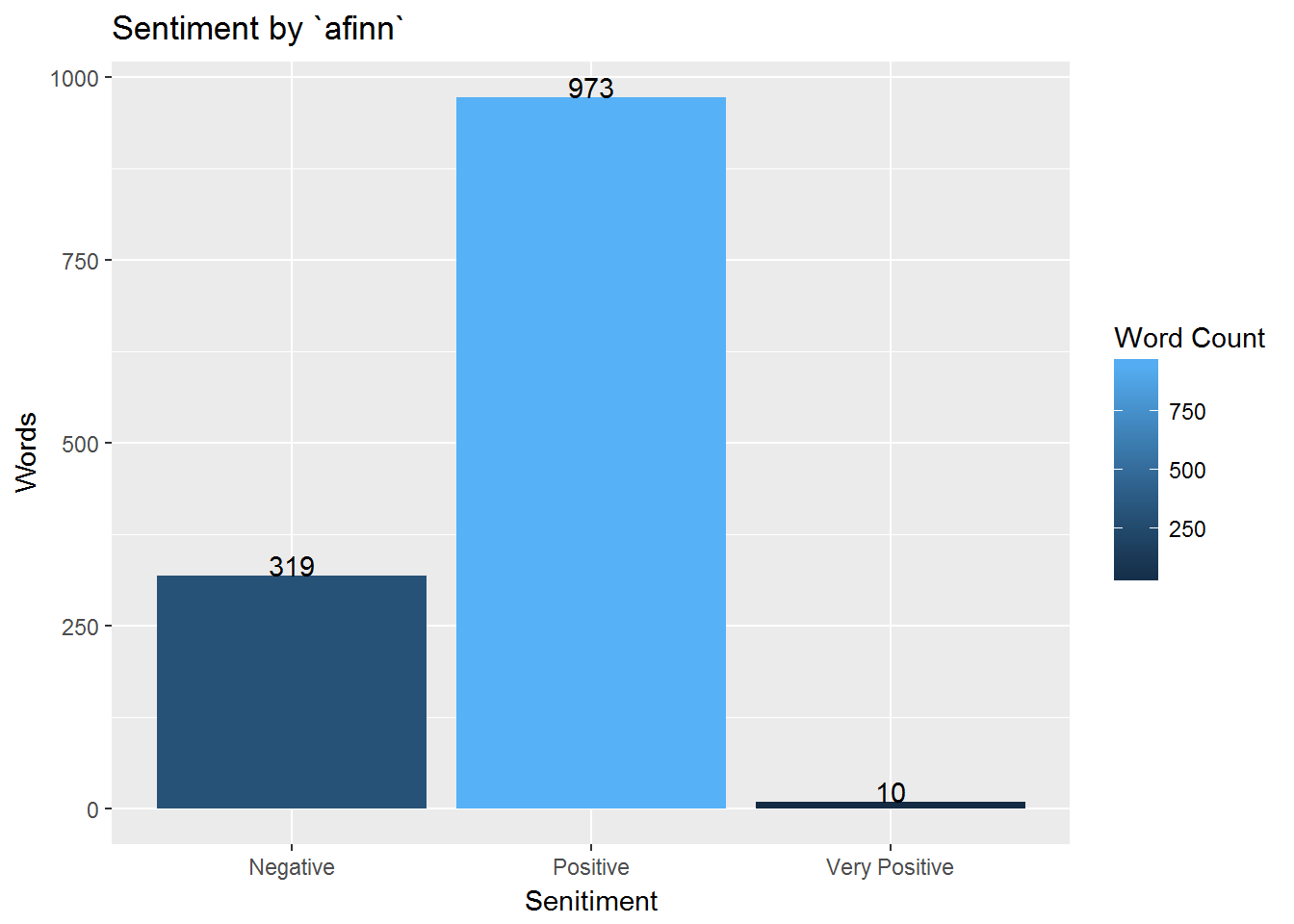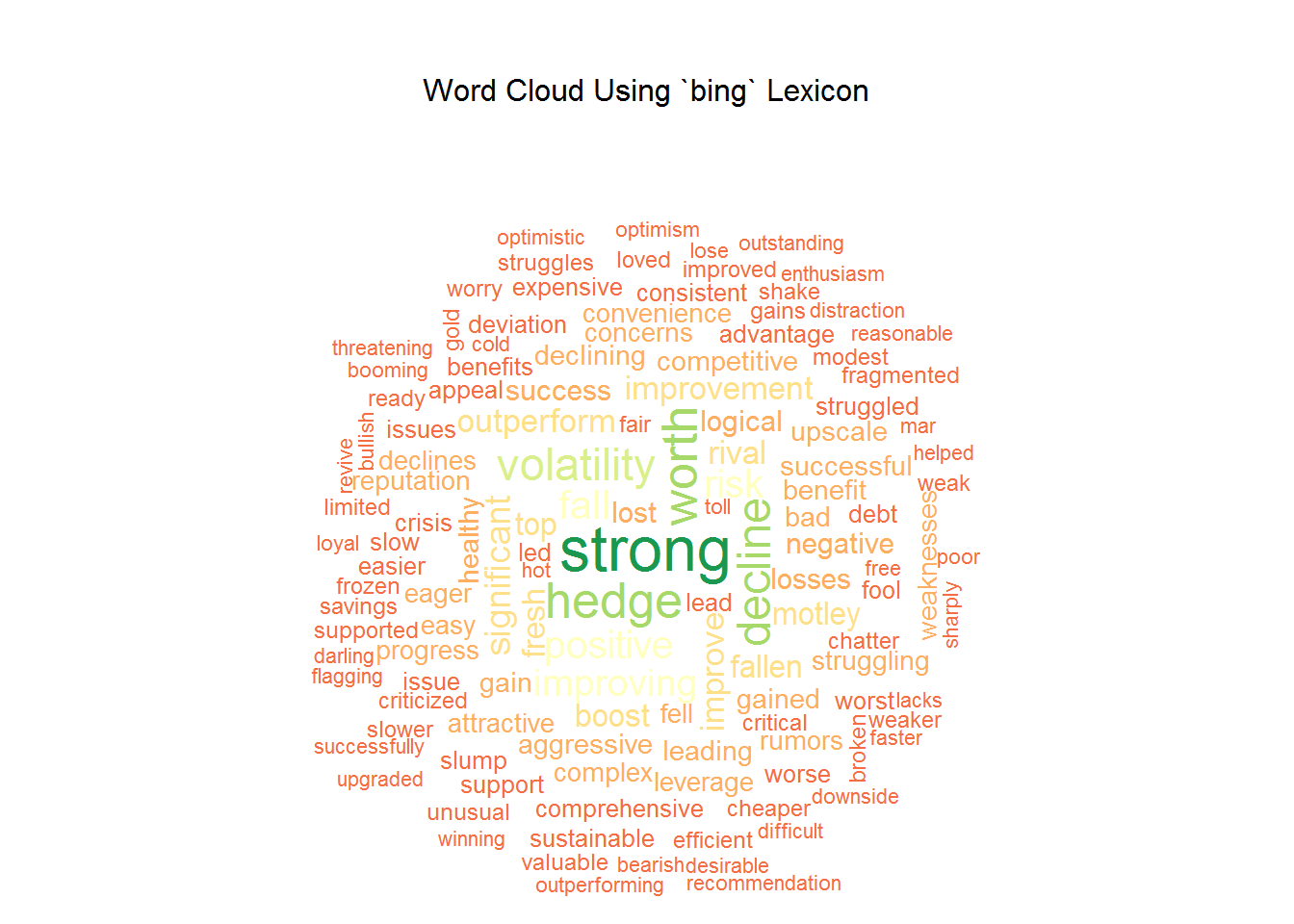## Abstract

The goal of this project is to perform Sentiment Analysis of news articles about Whole Foods Market company. At the beginning of April 2017 news outlets started publishing news, investors of privately-owned grocery chain Albertsons were considering the Whole Foods Market takeover. Some articles favored acquisition while others described the weakness in sales and revenues of the company.

## Introduction

As part of the project, I will be presenting multiple ways to perform Sentiment Analysis.

• Basic Sentiment Analysis
• Sentence Sentiment Analysis

There are many R packages available to perform Sentiment Analysis, for the scope of the project I will be using three packages `tidytext`, `sentimentr` and `syuzhet`. The main idea is to compare sentiment polarity scores based on `lexicon` and `package`. Finally, sentiment scores and stock prices will be combined to evaluate whether there is a linear relationship between `news sentiment` and `stock price movement`.

## Method and Software Usage

The principles of tidy data provided by `Hadley Wickham` are followed throughout the process of cleaning and preparing the data for analysis. The software tool used for the project is `R`. Basic sentiment analysis is done using three lexicons `afinn`, `bing` and `nrc` from `tidytext` package. Sentence Sentiment Analysis and Document Sentiment Analysis is done using `sentimentr` package. Function from technical trading rules `TTR` package is used for calculating of `Moving Averages` and `Volatality` of the stock price. `MongoDB` NoSQL database is used for storing data.

## Data Source

For sentiment analysis, all news articles are harvested manually by web scraping. Data harvested from each website is saved as the text file on the local drive and also loaded into `MongoDB` database for future usage. Using functions from `tm` package document `corpus` is created for processing. Whole Foods Market stock prices are imported from Yahoo! finance website using `quantmod` package.

#### Libraries used.

``````if (!require('syuzhet')) install.packages('syuzhet')          #Web scraping and text extraction
if (!require('plyr')) install.packages('plyr')                #Data frame and table functions
if (!require('dplyr')) install.packages('dplyr')              #Data frame and table functions
if (!require('stringr')) install.packages('stringr')          #String manuplilation functions
if (!require('tm')) install.packages('tm')                    #create document corpus and DocumentTermMatrix
if (!require('quantmod')) install.packages('quantmod')        #Get stock prrices
if (!require('TTR')) install.packages('TTR')                  #Calculate Moving Averages, RSI, Volatality
if (!require('tidyr')) install.packages('tidyr')              #Tidy data using spread() and gather() functions
if (!require('tidytext')) install.packages('tidytext')        #Word sentiment analysis
if (!require('sentimentr')) install.packages('sentimentr')    #Sentence sentiment analysis
if (!require('ggplot2')) install.packages('ggplot2')          #Graphics display
if (!require('gridExtra')) install.packages('gridExtra')      #Display graphs side by side
if (!require('wordcloud')) install.packages('wordcloud')      #Create word cloud
if (!require('RColorBrewer')) install.packages('RColorBrewer') #Get color palette
if (!require('knitr')) install.packages('knitr')              #Report display, table format
if (!require('quantmod')) install.packages('quantmod')        #Get stock prices
if (!require('jsonlite')) install.packages('jsonlite')        #converting data into JSON
if (!require('mongolite')) install.packages('mongolite')      #connecting to MongoDB``````

Load all articles and stock price data into `MongoDB` NoSQL database.

``````#Function to cleanup data
cleanData <- function(x){
x <- gsub(":", "", x) # Replace junk with ("")
x <- iconv(x, "latin1", "ASCII", sub=" ")
x <- gsub("\\s+", " ", x) # Remove double spaces
return(x)
}

properDate <- function(x){
y <- str_sub(x, -4) #Get last 4 digits
m <- substr(x,1,2)
d <- paste0(y, "-", m, "-", substr(x,3,4), " 00:00:00")
d <- as.Date(d)
return(d)
}

#Create mongoDB connection to load articles
mongoCon <- mongo(collection = "articles", db = "finalProject")
query = '{}'
fields = ' {"_id" : 0}'
mongo.article <- mongoCon\$find(query, fields)

#Articles does not exist in MongoDB insert them
if (nrow(mongo.article) < 1){
#Change path
rootDir = "D:/CUNY/607/FinalProject/Final/Articles/"
pattern = "txt\$"
fileFolder <- rootDir
fileList <- list.files(path = fileFolder, pattern = pattern, all.files = FALSE, full.names = TRUE, recursive = FALSE)

for(f in fileList){

#Read first line it contains source of the article
article.source <- article.text

#Get entire text from the file
article.text <- f %>% get_text_as_string()

#Cleanup text
article.text <- cleanData(article.text)

#remove source link from the article

#Article file name
article.fileName <- gsub(pattern = rootDir, replacement = "", f)

#Article date
pattern <- "[[:digit:]]+"
article.date <- properDate(str_extract(article.fileName, pattern))

artilce.df <- data.frame(article.fileName, article.source, article.date, article.text)
colnames(artilce.df) <- gsub("\\.", "_", colnames(artilce.df))

#Check if article already present in DB
query = paste0('{"article_fileName": "', article.fileName,'" }')
fields = ' {"_id" : 1, "article_source" : 1, "article_date" : 1, "article_text" : 1 }'
mongo.article <- mongoCon\$find(query, fields)

if(nrow(mongo.article) == 0){
#if file does not exist load it
mongoCon\$insert(artilce.df)
} else{
#Source changed update
if (!grepl(cleanData(mongo.article\$article_source), article.link, ignore.case = T)){

query = paste0('{"_id": { "\$oid" : "', mongo.article\$`_id`,'" } }')
update = paste0('{ "\$set" : { "article_source" : "', article.source, '"} }')

a<-mongoCon\$update(query, update)
}
}
}

query = '{}'
fields = ' {"_id" : 0}'
mongo.article <- mongoCon\$find(query, fields)

}

#Close connection
rm(mongoCon)

#Create mongoDB connection to load stock price
mongoCon <- mongo(collection = "stockPrice", db = "finalProject")

query = '{}'
fields = ' {"_id" : 0}'
mongo.stock.price <- mongoCon\$find(query, fields)

#Data does not exist connect to Yahoo finance and get data
if (nrow(mongo.stock.price) < 1){
#Obtain stock price, Whole Foods Market ticker is 'WFM'
wfm.stock.data <- new.env()
getSymbols('WFM',src='yahoo',env = wfm.stock.data)

#Convert to data frame
wfm.stock.data <-as.data.frame(wfm.stock.data\$WFM)
rownames(wfm.stock.data) <- NULL

#Add average stock price per day
wfm.stock.data <- wfm.stock.data %>% mutate(avg_stockprice = (WFM.Open + WFM.High + WFM.Low) / 3)

#Calculate moving averages
wfm.stock.data\$ma_10 <- round(SMA(wfm.stock.data\$WFM.Close, n = 10),2)
wfm.stock.data\$ma_50 <- round(SMA(wfm.stock.data\$WFM.Close, n = 50),2)

#Calculate exponential moving averages
wfm.stock.data\$ema_10 <- round(EMA(wfm.stock.data\$WFM.Close, n = 10),2)
wfm.stock.data\$ema_50 <- round(EMA(wfm.stock.data\$WFM.Close, n = 50),2)

#Volatility data
Vol <- data.frame(Vol = volatility(OHLC(wfm.stock.data), n = 10, N = 260, mean0 = FALSE, calc="garman"))
wfm.stock.data <- cbind(wfm.stock.data,vol_garman=Vol\$Vol)

mongoCon\$insert(wfm.stock.data)

query = '{}'
fields = ' {"_id" : 0}'
mongo.stock.price <- mongoCon\$find(query, fields)
}

#Close connection
rm(mongoCon)``````

#### Corpus, Term Document Matrix(TDM) and Sentences Generation

Text inside each articles is broken into words using `TermDocumentMatrix` function from `tm` package. Also, text is seperated into sentences using `unnest_tokens` function from `tidytext` package.

``````#Read files and create Term Document Matrix
filesToTDM <- function(x){
#Generate corpus for filelist

#Clean up the corpus
wfm.article.corpus <- tm_map(wfm.article.corpus, removePunctuation)
wfm.article.corpus <- tm_map(wfm.article.corpus, removeNumbers)
wfm.article.corpus <- tm_map(wfm.article.corpus, stripWhitespace)
wfm.article.corpus <- tm_map(wfm.article.corpus, content_transformer(tolower))
wfm.article.corpus <- tm_map(wfm.article.corpus, PlainTextDocument)

#Generate Document Term Matrix
wfm.tdm <- TermDocumentMatrix(wfm.article.corpus)
tdmOutput <- list(tdm = wfm.tdm, articleFile = x\$article_fileName)
return(tdmOutput)
}

#Function to cleanup data
cleanData <- function(x){
x <- ifelse(grepl("http", x), NA, x)
x <- gsub("[[:punct:]]", "", x) # Replace junk with ("")
x <- iconv(x, "latin1", "ASCII", sub=" ")
x <- gsub("\\s+", " ", x) # Remove double spaces
return(x)
}

properDate <- function(x){
y <- str_sub(x, -4) #Get last 4 digits
m <- substr(x,1,2)
d <- paste0(y, "-", m, "-", substr(x,3,4), " 00:00:00")
d <- as.Date(d)
return(d)
}

getSentenceFromText <- function(x){
#Create empty table to store sentences from articles
articles.sentence.df <- data.frame(sentence<-NA, filename<-NA, stringsAsFactors = F)
colnames(articles.sentence.df) <- c("sentence", "fileName")

for(i in 1:nrow(x)){

#Get entire text from the file
fileText <- x\$article_text[i]

#Tibble is used as unnest_tokens expects data in table format
textToSentence <- tibble(text = fileText) %>% unnest_tokens(sentence, text, token = "sentences") %>% as.data.frame(stringsAsFactors = F)
textToSentence\$fileName <- x\$article_fileName[i]
textToSentence\$sentence <- cleanData(textToSentence\$sentence)

articles.sentence.df <- rbind(articles.sentence.df, textToSentence)
}
articles.sentence.df <- na.omit(articles.sentence.df)
rownames(articles.sentence.df) <- NULL
return(articles.sentence.df)
}

#Convert files to Document Term Matrix
wfm.articles.tdm <- filesToTDM(mongo.article)
wfm.articles.matrix <- data.matrix(wfm.articles.tdm[["tdm"]])

#Convert matrix to dataframe
wfm.articles.df <- as.data.frame(wfm.articles.matrix, stringsAsFactors = F)

wfm.articles.filename <- wfm.articles.tdm[["articleFile"]]

#Bind filenames to columns
colnames(wfm.articles.df) <- wfm.articles.filename

#Clean data get words from rownames
wfm.articles.df\$fileWords <- cleanData(rownames(wfm.articles.df))
rownames(wfm.articles.df) <- NULL

#Transpose columns to rows
wfm.tidy.data <- gather(wfm.articles.df, fileWords)
colnames(wfm.tidy.data) <- c("fileWord","fileName","wordCount")

#Ignore rows with NA values and wordCount less than 1. Means word does not exist in the article
wfm.tidy.data <- na.omit(wfm.tidy.data)
wfm.tidy.data <- wfm.tidy.data[wfm.tidy.data\$wordCount>0, ]
rownames(wfm.tidy.data) <- NULL

#Get stop words from 'tidytext' package and remove from data frame
lexStopWords <- stop_words
wfm.tidy.data <- wfm.tidy.data %>%
anti_join(lexStopWords  , by = c("fileWord" = "word")) %>%
filter(!fileWord  %in% c("april", "byteresa", "cfra", "jana","npd", "shopjana","wfm","ihor","amazoncom","anayahooyptryahoo","bloomberg","carolinabased","cincinnatibased","cincinnati", "monday", "month","dusaniwsky"))

#Get sentences from each text file
#This data frame will used for sentence analysis
wfm.articles.sentence.df <- getSentenceFromText(mongo.article)

#Attach date
pattern <- "[[:digit:]]+"
wfm.articles.sentence.df\$articleDate <- properDate(str_extract(wfm.articles.sentence.df\$fileName, pattern))
wfm.tidy.data\$articleDate <- properDate(str_extract(wfm.tidy.data\$fileName, pattern))``````

### Basic Sentiment Analysis

This is a simple sentiment analysis focusing only at the word level. The `tidytext` package contains three sentiment lexicons. All three lexicons are based on unigrams and contain many English words.

• `bing` by Bing Liu, classifies words from each sentence into positive and negative sentiment.
• `nrc` by Saif Mohammad and Peter Turney, classifies words into emotions like positive, negative, anger, anticipation, disgust, fear, joy, sadness, surprise, and trust.
• `afinn` by Finn Arup Nielsen, assigns words with a score that runs between -5 and 5, with negative scores indicating negative sentiment and positive scores indicating positive sentiment.

`bing` lexicon.

``````#Get words from bing lexicon
bingLexWord <- get_sentiments("bing")

#Apply sentiment to words
wfm.bing <- wfm.tidy.data %>% inner_join(bingLexWord, by = c("fileWord" = "word"))``````

`nrc` lexicon.

``````#Get words from bing lexicon
nrcLexWord <- get_sentiments("nrc")

#Apply sentiment to words
wfm.nrc <- wfm.tidy.data %>% inner_join(nrcLexWord, by = c("fileWord" = "word"))``````

`afinn` lexicon.

``````#Get words from bing lexicon
afinnLexWord <- get_sentiments("afinn")

#Apply sentiment to words
wfm.afinn <- wfm.tidy.data %>% inner_join(afinnLexWord, by = c("fileWord" = "word"))``````

### Comparing Lexicons

Following graphs show Sentiment score assigned by each lexicon to individual words in all documents in the corpus.